Case Based Questions (MCQ)

Chapter 2 Class 10 Polynomials
Serial order wise

## An asana is a body posture, originally and still a general term for a sitting meditation pose, and later extended in hatha yoga and modern yoga as exercise, to any type of pose or position, adding reclining, standing, inverted, twisting, and balancing poses. In the figure, one can observe that poses can be related to representation of quadratic polynomial.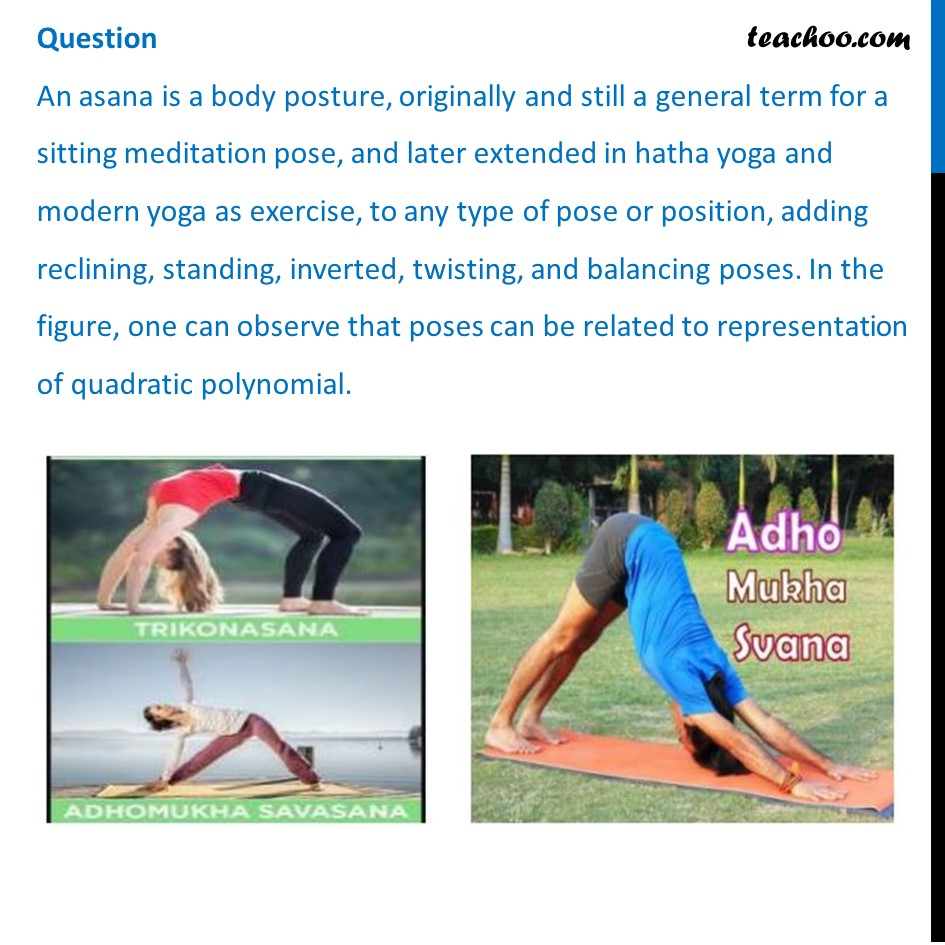## The shape of the poses shown is (a) Spiral  (b) Ellipse  (c) Linear  (d) Parabola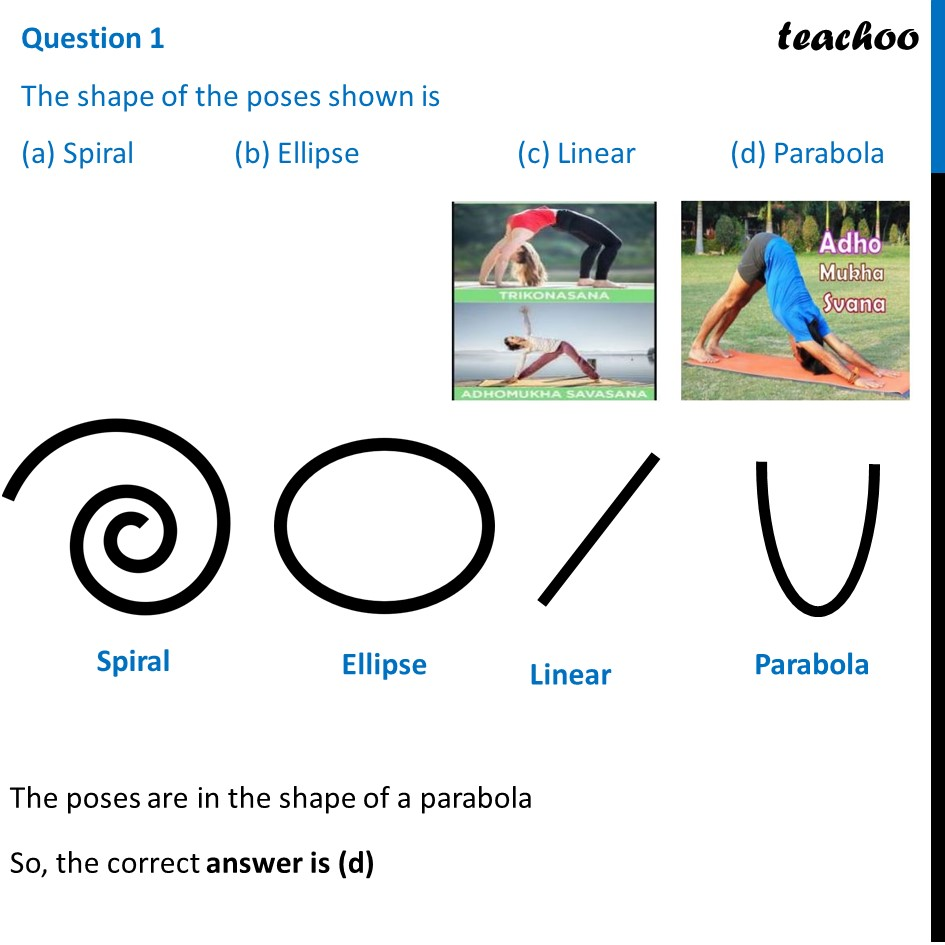## The graph of parabola opens downwards, if _______ (a) a ≥ 0  (b) a = 0   (c) a < 0   (d) a > 0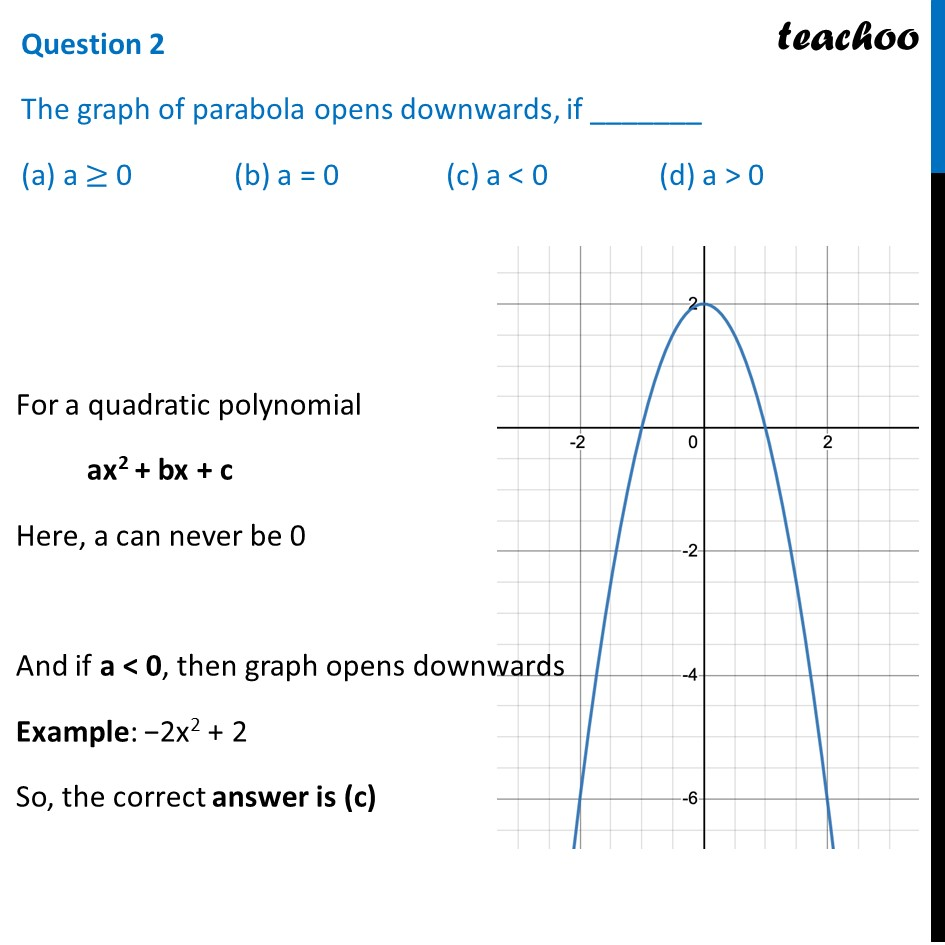## In the graph, how many zeroes are there for the polynomial? (a) 0  (b) 1  (c) 2  (d) 3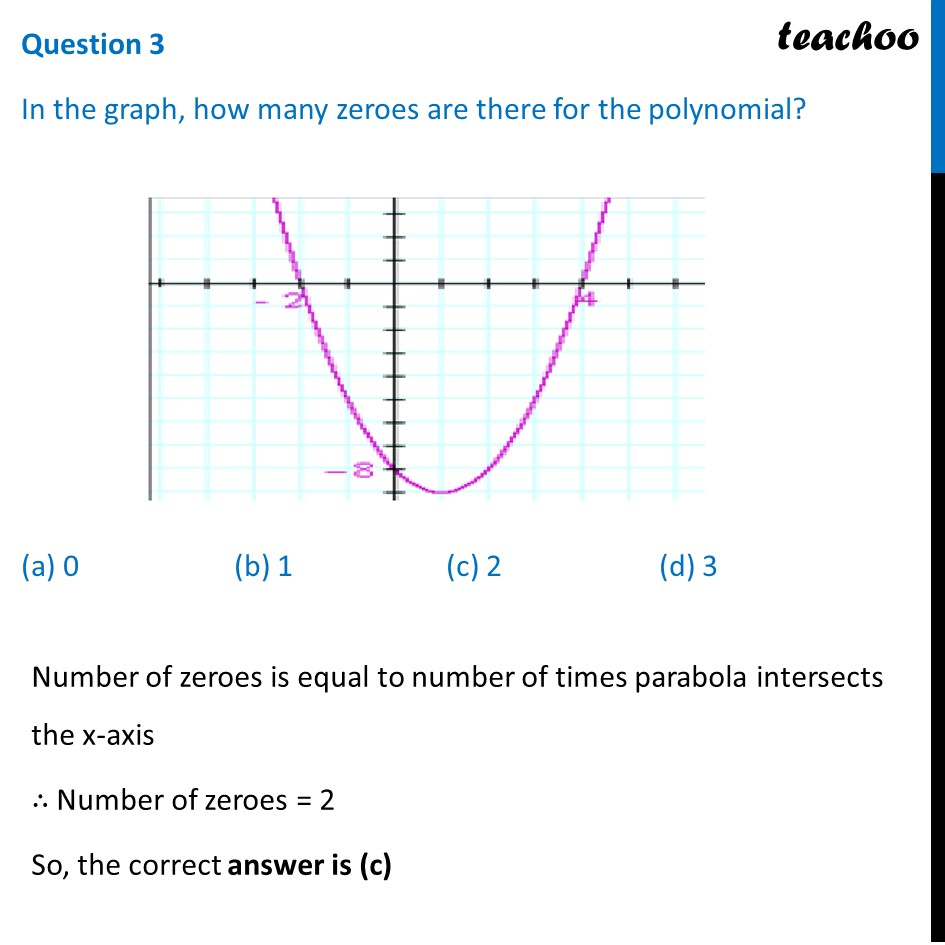## The two zeroes in the above shown graph are (a) 2, 4   (b) –2, 4  (c) –8, 4   (d) 2, –8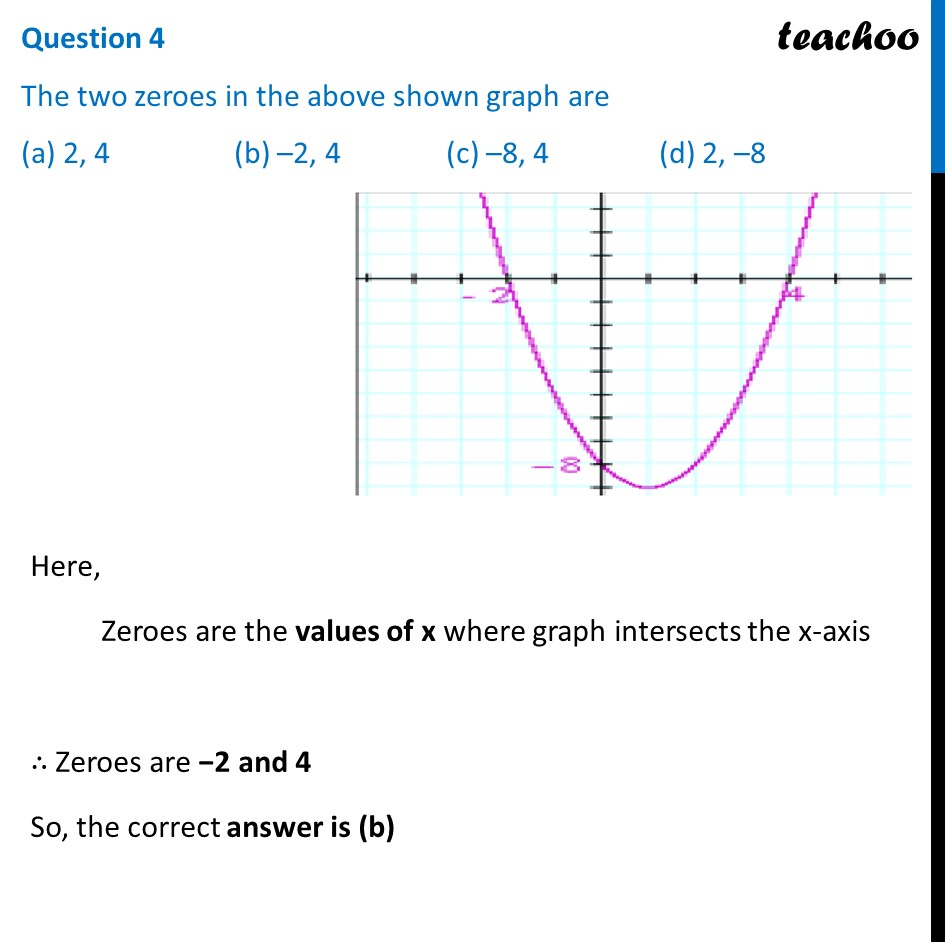## (a) 2/√3,√3/4 (b) (−2)/√3,√3/4 (c) 2/√3,(−√3)/4 (d) (−2)/√3,(−√3)/4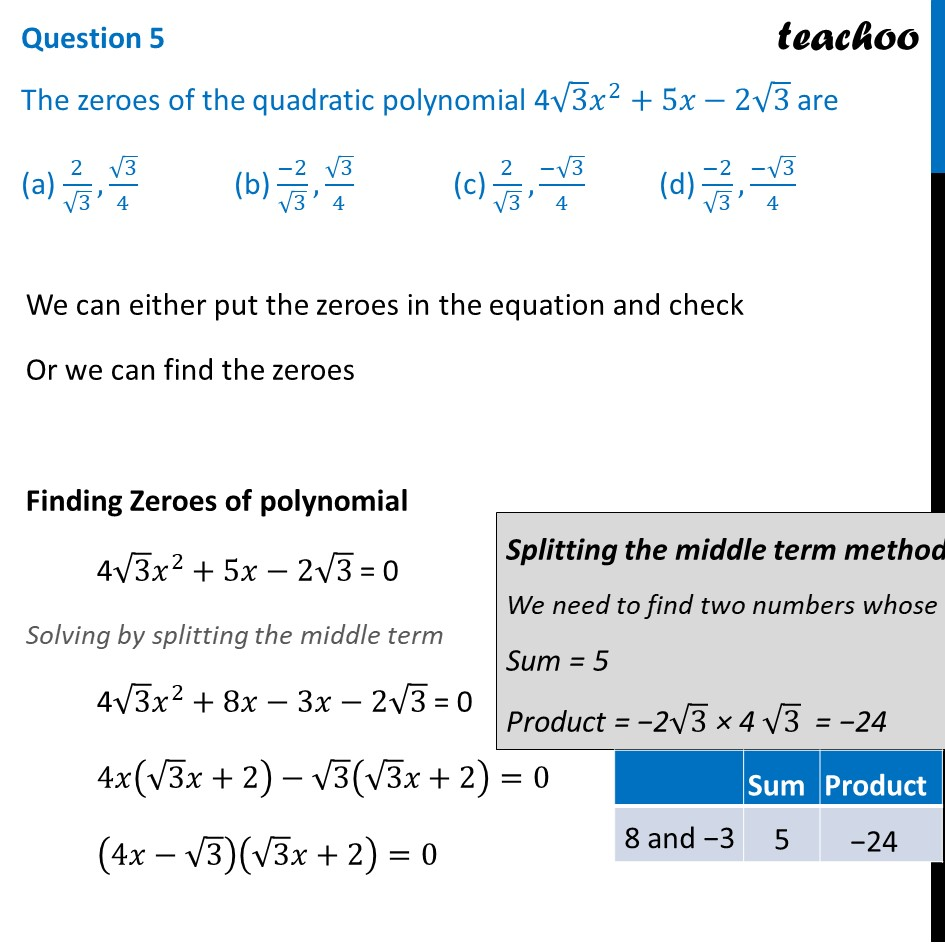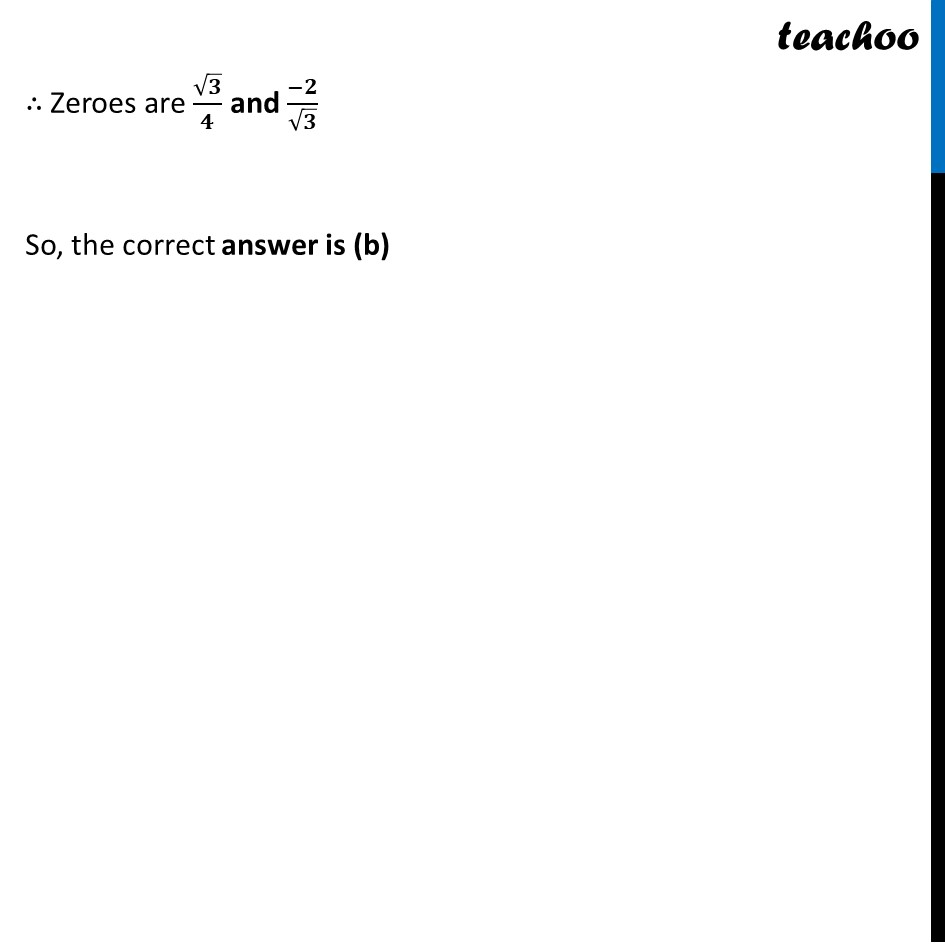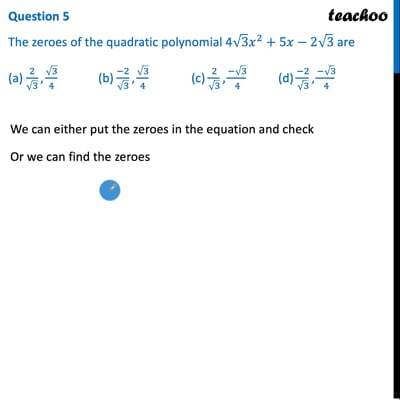This video is only available for Teachoo black users

Introducing your new favourite teacher - Teachoo Black, at only ₹83 per month

### Transcript

Question An asana is a body posture, originally and still a general term for a sitting meditation pose, and later extended in hatha yoga and modern yoga as exercise, to any type of pose or position, adding reclining, standing, inverted, twisting, and balancing poses. In the figure, one can observe that poses can be related to representation of quadratic polynomial.Question 1 The shape of the poses shown is (a) Spiral (b) Ellipse (c) Linear (d) Parabola The poses are in the shape of a parabola So, the correct answer is (d) Question 2 The graph of parabola opens downwards, if _______ (a) a ≥ 0 (b) a = 0 (c) a < 0 (d) a > 0 For a quadratic polynomial ax2 + bx + c Here, a can never be 0 And if a < 0, then graph opens downwards Example: −2x2 + 2 So, the correct answer is (c) Question 3 In the graph, how many zeroes are there for the polynomial? (a) 0 (b) 1 (c) 2 (d) 3 Number of zeroes is equal to number of times parabola intersects the x-axis ∴ Number of zeroes = 2 So, the correct answer is (c) Question 4 The two zeroes in the above shown graph are (a) 2, 4 (b) –2, 4 (c) –8, 4 (d) 2, –8 Here, Zeroes are the values of x where graph intersects the x-axis ∴ Zeroes are −2 and 4 So, the correct answer is (b) Question 5 The zeroes of the quadratic polynomial 4√3 𝑥^2+5𝑥−2√3 are (a) 2/√3,√3/4 (b) (−2)/√3,√3/4 (c) 2/√3,(−√3)/4 (d) (−2)/√3,(−√3)/4 We can either put the zeroes in the equation and check Or we can find the zeroes Finding Zeroes of polynomial 4√3 𝑥^2+5𝑥−2√3 = 0 Solving by splitting the middle term 4√3 𝑥^2+8𝑥−3𝑥−2√3 = 0 4𝑥(√3 𝑥+2)−√3 (√3 𝑥+2)=0 (4𝑥−√3)(√3 𝑥+2)=0 ∴ Zeroes are √𝟑/𝟒 and (−𝟐)/√𝟑 So, the correct answer is (b)